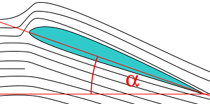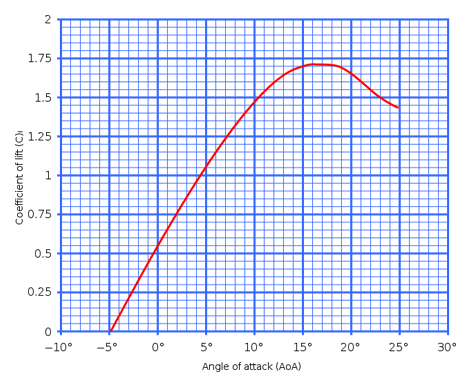Angle of attackBy Wikipedia,
the free encyclopedia,

http://en.wikipedia.org/wiki/Angle_of_attackIn this diagram, the black lines represent the flow of a fluid around a two-dimensional airfoil shape. The angle α is the angle of attack.

Angle of attack (AOA, α, Greek letter alpha) is a term used in fluid dynamics to describe the angle between a reference line on a body (often the chord line of an airfoil) and the vector representing the relative motion between the body and the fluid through which it is moving. In general, the reference line could be any line on any arbitrarily shaped body in a flow. The angle of attack would be the angle between the line and the oncoming flow. This article focuses on the most common application, the angle of attack of a wing or airfoil moving through air. For a two-dimensional airfoil, angle of attack is the angle between the chord line and the direction of arrival of the incoming fluid.

In aviation, angle of attack is used to describe the angle between the chord line of the (three dimensional) wing of a fixed-wing aircraft and the vector representing the relative motion between the aircraft and the atmosphere. Since a wing can have twist, a chord line of the whole wing may not be definable, so an alternate reference line is simply defined. Often, the chord line of the root of the wing is chosen as the reference line. Another alternative is to use a horizontal line on the fuselage as the reference line (and also as the longitudinal axis).

In traditional British usage, the term angle of incidence is used instead of angle of attack.

## Confusion between angle of attack and pitch angle

The angle of attack is often confused with the pitch angle of an aircraft. Pitch angle is measured from the same reference line as angle of attack (conceptually the chord line), but to the horizon. Whereas, the angle of attack is measured from the (same) reference line to the direction of the arrival of the air to the aircraft/wing (caused by the relative motion between the aircraft/wing and the atmosphere).

In straight and level flight (through air that is not rising or falling), pitch angle and angle of attack have the same values. In this situation, the air (the relative wind) happens to be arriving toward the aircraft from the direction of the horizon, and so the two angles are the same in size. In straight descending flight, angle of attack becomes greater than pitch angle because the air arrives at the aircraft from below the horizon. Conversely, in straight ascending flight, angle of attack becomes less than pitch angle.

## Relation to Angle of sideslip

Angle of sideslip is similar to angle of attack. For a three-dimensional body like an airplane, it measures the angle of arrival of the oncoming air off the side of the nose, while angle of attack measures the angle of the oncoming air below the nose.

## Relation between angle of attack and liftA typical lift coefficient curve.

The lift coefficient of a fixed-wing aircraft is directly related to the angle of attack. Increasing angle of attack is associated with increasing lift coefficient up to the maximum lift coefficient, after which lift coefficient decreases.

As the angle of attack on the wing of a fixed-wing aircraft increases, separation of the airflow from the upper surface of the wing becomes more pronounced, leading to a reduction in the rate of increase of the lift coefficient.

#### Critical angle of attack

The critical angle of attack is the angle of attack which produces the maximum lift. This is also called the "stall angle of attack". Below the critical angle of attack, as the angle of attack increases, the coefficient of lift (Cl) increases. At the same time, below the critical angle of attack, as angle of attack increases, the air begins to flow less smoothly over the upper surface of the airfoil and begins to separate from the upper surface. On most airfoil shapes, as the angle of attack increases, the upper surface separation point of the flow moves forward from the trailing edge towards the leading edge. At the critical angle of attack, upper surface flow is more separated and the airfoil or wing is producing its maximum lift for the airspeed. As angle of attack increases further, the upper surface flow becomes more and more fully separated and the airfoil/wing produces less lift.

Above this critical (maximum-lift) angle of attack, the aircraft is said to be in a stall. A fixed-wing aircraft is stalled at or above the critical angle of attack rather than at or below a particular airspeed. The airspeed at which the aircraft stalls is variable, depending on the weight of the aircraft, the load factor at the time and the thrust from the engine. The critical angle of attack is typically around 15° for many airfoils.

Some aircraft are equipped with a built-in flight computer that automatically prevents the aircraft from increasing the angle of attack any further when the maximum angle of attack is reached, irrespective of pilot input. This is called the 'angle of attack limiter' or 'alpha limiter'. Modern airliners that have fly-by-wire technology avoid the critical angle of attack by means of software in the computer systems that govern the flight controls.

When takeoff and landing operations are critical, such as Naval Aircraft Carrier operations and STOL back country flying, aircraft may be equipped with angle of attack or Lift Reserve indicators. These indicators measure the angle of attack (AOA) or the Potential of Wing Lift (POWL, or Lift Reserve) directly and help the pilot fly these operations close to the stall point with greater precision. STOL operations require the aircraft to be able to operate at full-stall during landings and maximum climb angle during takeoffs. Since the airspeed of maximum performance during these maneuvers varies, airspeed information is of less value to the pilot than AOA information.

## Very high alpha

In some military aircraft that do not display a conventional critical angle of attack, the aircraft is able to achieve a very high angle of attack. This provides the aircraft with great agility. A famous military example is Pugachev's Cobra.

Using a variety of additional aerodynamic surfaces — known as high-lift devices — like leading edge extensions (leading edge wing root extensions), fighter aircraft have increased the potential flyable alpha from about 20° to over 45°..

## Local Angle of Attack

While angle of attack of an aircraft is strictly defined as the angle between a reference line and the direction of arrival of the far upstream oncoming flow, the directions of the flow change as it passes around the body. For many aerodynamic surfaces on an aircraft, the "far-enough upstream" flow may have already been deflected by other parts of the aircraft. This sets up a local oncoming flow direction that is slightly different than the oncoming flow far upstream of the whole aircraft. The "local angle of attack" is the angle between the chord line of the surface (or other reference line) and the local upstream oncoming flow.

For example, the wing produces downwash. Downwash redirects the flow behind the wing downward. The horizontal tail sees this slightly downward-angled flow as its oncoming flow, so the local angle of attack for the horizontal tail is less than the angle of attack of the whole aircraft (assuming the same reference line used for both the aircraft and tail).

## Sailing

In sailing, the angle of attack is the angle between a mid-sail and the direction of the wind. The physical principles involved are the same as for aircraft. See points of sail.

1. ^ Gracey, William (1958). "Summary of Methods of Measuring Angle of Attack on Aircraft". NACA Technical Note (NASA Technical Reports) (NACA-TN-4351): 1-30.
2. ^ Wikipedia: High Alpha Research Vehicle

Published in July 2009.